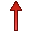# A5 Card

This card specifies excitation by an electric Hertzian dipole

On the Source/Load tab, in the Ideal sources group, click theElectric dipole (A5) icon.

## Parameters:

New source
A new excitation is defined which replaces all previously defined excitations.
A new excitation is defined which is added to the previously defined excitations.
Magnitude of I dl (A m)
Absolute value of the complex amplitude $I\cdot l$ in Am.
Phase of I dl (degrees)
Phase of the complex amplitude $I\cdot l$ in degrees.
X, Y, Z position
Coordinates of the position of the dipole in m. These values are optionally scaled by the SF card.
$\vartheta$ angle
Orientation of the dipole in space. The angle $\vartheta$ (in degrees) is the angle between the dipole and the Z axis.
$\phi$ angle
Orientation of the dipole in space. The angle $\phi$ (in degrees) is the angle between the projection of the dipole onto the Z=0 and the X axis.
The dipole moment of the electric dipole is given by
(1) $p=\frac{Il}{j\omega }$

The power radiated by the dipole in a free space environment is given by

(2) $p=\frac{{\beta }_{0}^{2}{Z}_{F0}{|Il|}^{2}}{12\pi }=\frac{{\omega }^{2}{µ}_{0}^{2}{|Il|}^{2}}{12\pi {Z}_{F0}}$

Feko, however, considers the properties of the medium in which the dipole is located, as well as the coupling of the dipole with surrounding structures or other sources (for example other Hertzian dipoles in an array antenna), when calculating the power radiated by the Hertzian dipole.